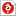검색어 입력폼

# 성균관대 진동및동적시스템설계실습 질점낙하 Point Mass Downfall

저작시기 2014.10 | 등록일 2015.03.08한컴오피스 (hwp) | 15페이지 | 가격 1,500원

## 목차

1. Point Mass Downfall
1.1 Objective of experiment
1.2 Basic theory
1.3 Experimental procedure
1.4 Result
1.5 Discussion

2. The Center of Mass and Mass Moment of Inertia
2.1 Objective of experiment
2.2 Basic theory
2.3 Experimental procedure
2.4 Result
2.5 Discussion

3. Reference

## 본문내용

1. Point Mass Downfall
1.1 Objective of experiment
The object falling on the curvature is off-bending in a moment and goes the free-fall. In theory, calculate the falling point of object and review the results as compared to the measuring values.

1.2 Basic theory
1.2.1 Conservation of mechanical energy
According to the principle of conservation of mechanical energy, the mechanical energy of an isolated system remains constant in time, as long as the system is free of friction and other non-conservative forces. In other words, if there are no nonconservative forces, then no nonconservative work is done and mechanical energy is conserved.

## 참고 자료

http://en.wikipedia.org/wiki/Mechanical_energy#cite_note-Access_CL-1
Benson H. Tongue, Dynamics Engineering Mechanics, Second Edition, WILEY
http://physics.bu.edu/~okctsui/PY105%20Lecture_notes/Notes/class30_Rotational_KE.pdf
http://hyperphysics.phy-astr.gsu.edu/hbase/cm.html
http://en.wikipedia.org/wiki/Moment_of_inertia
http://gauss.vaniercollege.qc.ca/pwiki/index.php/Rotational_Kinetic_Energy_and_Moment_Of_Inertia
http://hyperphysics.phy-astr.gsu.edu/hbase/parax.html